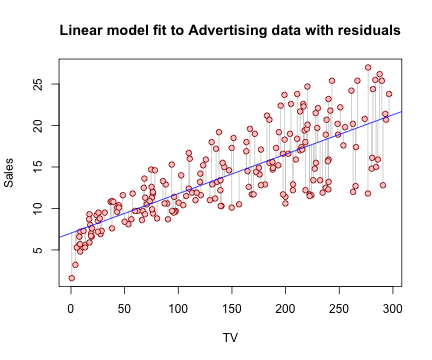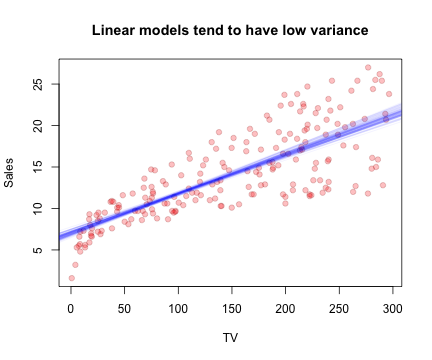## Thursday, February 06, 2014

### Linear Models

Notes on chapter 3 of Introduction to Statistical Learning and the Stanford Online Statistical Learning class.

The chapter uses the Advertising data set available from the book's website:

Advertising <- read.csv("http://www-bcf.usc.edu/~gareth/ISL/Advertising.csv",
row.names = 1)


A linear model has the form $$Y = \beta_0 + \beta_1 X$$. The task of linear regression is to estimate the betas from the data. With the estimated coefficients, we can estimate the response, $$\hat{y}_i$$, produced by a given predicter, $$x_i$$, by $$\hat{y}_i = \hat{\beta}_0 + \hat{\beta}_1 x$$.

#### Fitting a linear model

Estimating the coefficients: $\hat{\beta}_1 = \frac{\sum_{i=1}^n (x_i-\bar{x})(y_i-\bar{y})}{\sum_{i=1}^n (x_i-\bar{x})^2}$ $\hat{\beta}_0 = \bar{y} - \hat{\beta}_1 \bar{x}$

For the Advertising data, this can be computed in code like so:

X <- Advertising$TV Y <- Advertising$Sales
x_bar <- mean(X)
y_bar <- mean(Y)
beta_hat_1 <- sum((X - x_bar) * (Y - y_bar))/sum((X - x_bar)^2)
beta_hat_0 <- y_bar - beta_hat_1 * x_bar
cat(beta_hat_0, beta_hat_1)

## 7.033 0.04754


Luckily, R provides the lm function.

fit <- lm(Sales ~ TV, data = Advertising)
coef(fit)

## (Intercept)          TV
##     7.03259     0.04754


Reproducing figure 3.1 from the book shows sales plotted against TV advertising. The regression line is overlayed in blue and the vertical line segments are the residuals at each data point.

plot(Sales ~ TV, data = Advertising, type = "n")
segments(Advertising$TV, Advertising$Sales, Advertising$TV, predict(fit, Advertising), col = "#cccccc") points(Sales ~ TV, data = Advertising, pch = 21, col = "#990000", bg = "#ffcccc") abline(fit, col = "blue") title("Linear model fit to Advertising data with residuals")#### Assessing Accuracy How close an approximation of the true relationship is our model? ##### Standard Error of the Coefficients To compute standard error, we need to know $$\sigma^2$$, the variance of $$\epsilon$$. This can be approximated by the residual standard error $$RSE = \sqrt{RSS/(n-2)}$$ where RSS, the residual sum of squares, is defined as $$RSS = \sum_{i=1}^n (y_i - \hat{y}_i)^2$$. $SE(\hat{\beta}_0)^2 = \sigma^2 \left( \frac{1}{n} + \frac{\bar{x}^2}{\sum_{i=1}^n(x_i-\bar{x})^2} \right)$ $SE(\hat{\beta}_1)^2 = \frac{\sigma^2}{\sum_{i=1}^n(x_i-\bar{x})^2}$ The 95% confidence interval for the coefficients is plus or minus 2 standard errors $$\hat{\beta}_0 \pm 2 \cdot SE(\hat{\beta}_0)$$ and $$\hat{\beta}_1 \pm 2 \cdot SE(\hat{\beta}_1)$$. n = nrow(Advertising) y_hat = predict(fit, Advertising) rss = sum((Advertising$Sales - y_hat)^2)
cat("RSE=", sqrt(rse_squared))

## RSE= 3.259

se_beta_0 = sqrt(rse_squared * (1/n + (x_bar^2)/sum((X - x_bar)^2)))
se_beta_1 = sqrt(rse_squared/sum((X - x_bar)^2))
cat(se_beta_0, se_beta_1)

## 0.4578 0.002691


The standard errors of the betas can also be found in the output of summary for an lm object:

summary(fit)

##
## Call:
## lm(formula = Sales ~ TV, data = Advertising)
##
## Residuals:
##    Min     1Q Median     3Q    Max
## -8.386 -1.955 -0.191  2.067  7.212
##
## Coefficients:
##             Estimate Std. Error t value Pr(>|t|)
## (Intercept)  7.03259    0.45784    15.4   <2e-16 ***
## TV           0.04754    0.00269    17.7   <2e-16 ***
## ---
## Signif. codes:  0 '***' 0.001 '**' 0.01 '*' 0.05 '.' 0.1 ' ' 1
##
## Residual standard error: 3.26 on 198 degrees of freedom
## Multiple R-squared:  0.612,  Adjusted R-squared:  0.61
## F-statistic:  312 on 1 and 198 DF,  p-value: <2e-16


The 68–95–99.7 rule says, for normal distributions, 95% of the probability density falls within 2 standard deviations of the mean. Using that, the 95% confidence interval can be roughly approximated for the two coefficients.

c(beta_hat_0 - 2 * se_beta_0, beta_hat_0 + 2 * se_beta_0)

##  6.117 7.948

c(beta_hat_1 - 2 * se_beta_1, beta_hat_1 + 2 * se_beta_1)

##  0.04216 0.05292


This, too, can be computed from the output of lm.

confint(fit)

##               2.5 %  97.5 %
## (Intercept) 6.12972 7.93547
## TV          0.04223 0.05284


Turning regression into a hypothesis test is done through the t-statistic.

$t = \frac{\hat{\beta}_i - \beta_{null}}{SE(\hat{\beta}_i)}$

I'm a bit hazy on how to go from the t-statistic to a p-value, but it likely involves the pt function. Here's my best guess:

t.statistic = beta_hat_0/se_beta_0
p.value = 2 * pt(t.statistic, lower.tail = F, df = n - 2)
cat(t.statistic, p.value)

## 15.36 1.406e-35

##### Linear models have low variance

Here we're fitting 100 models from different random subsamples of the Advertising data. The lines fit to each subsample tend to be fairly similar.

plot(Sales ~ TV, data = Advertising, type = "n")
points(Sales ~ TV, data = Advertising, pch = 21, col = "#99000040", bg = "#ff000040")
for (i in 1:100) {
2/3), ])
abline(fit, col = "#0000ff10")
}
title("Linear models tend to have low variance")##### R2 Statistic

Recall from earlier, the residual standard error and the residual sum of squares:

$RSE = \sqrt{ \frac{\sum_{i=1}^n (y_i - \hat{y}_i)^2}{(n-2)} } = \sqrt{ \frac{RSS}{(n-2)} }$ $RSS = \sum_{i=1}^n (y_i - \hat{y}_i)^2$

The RSE provides a measure of lack of fit in units of the response. The R2 statistic also measures lack of fit but does so on a fixed scale between 0 and 1, giving the proportion of variance explained by the model.

$TSS = \sum_i (y_i-\bar{y})^2$ $R^2 = \frac{TSS-RSS}{TSS} = 1 - \frac{RSS}{TSS}$

The book talks about a relationship between R2 and correlation, but I have to think about that more.